# CBSE Class 5 Mathematics Introduction to Algebra MCQs

## Introduction to Algebra Class 5 Mathematics MCQ

Class 5 Mathematics students should refer to the following multiple-choice questions with answers for Introduction to Algebra in standard 5. These MCQ questions with answers for Grade 5 Mathematics will come in exams and help you to score good marks

### Introduction to Algebra MCQ Questions Class 5 Mathematics with Answers

• a) ( x +5)
• b) x 5
• c) 5 x

• a) 12 x
• b) 12 + x
• c) x - 12

#### Question: Sum of a and b is divided by 5

• a) a+b/5
• b) a/5 + b
• c) None of these

• a) m/3n
• b) 3m/n
• c) 3n/m

#### Question: Five times x - six times z =

• a) 5 x - 6 z
• b) 6 x - 5 z
• c) x - 5 z

Answer: 5 x - 6 z

• a) 3 x + 6
• b) 6 x + 3
• c) x + 7

#### Question: Quotient of x and y increased by?

• a)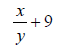• b)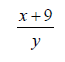• c)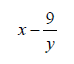• a) n + 12
• b) 12 - n
• c) 12 n

#### Question: Three times x minus five times x =

• a) 3 x - 5 y
• b) 5 x - 3 y
• c) x - 3 y

Answer: 3 x - 5 y

• a) 3(d + e)
• b) d + 3e
• c) 3 d + e

• a) 2a/3b
• b) 2a/b
• c) 2a/4b

• a) 12
• b) 2
• c) 13

• a) 2
• b) 6
• c) 4

• a) 20
• b) 4
• c) 24

#### Question: p =1/6, q=10 then p x q =?

• a) 5/3
• b) 5/6
• c) None of these

• a) 3
• b) 12
• c) 6

• a) 5
• b) 4
• c) 6

• a) 7
• b) 13
• c) 4

#### Question: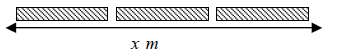Length of each part = ?

• a) x/3
• b) x-3
• c) 3x

#### Question: One half the product of base b and height h = ?

• a)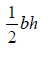• b)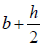• c) 2bh#### Question: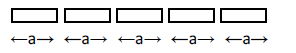Total Length = ?

• a) 5 a
• b) 5 + a
• c) a + 5

#### Question: x + 3 = 10, x represents ___________.

• a) known number
• b) unknown number
• c) none of these

#### Question: x + 3, x is known as

• a) variable
• b) none of these
• c) alphabet

• a) 3
• b) 9
• c) 12